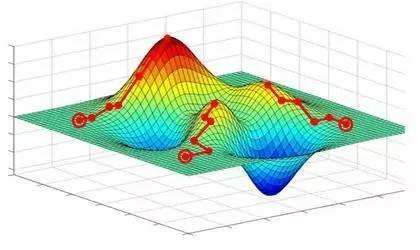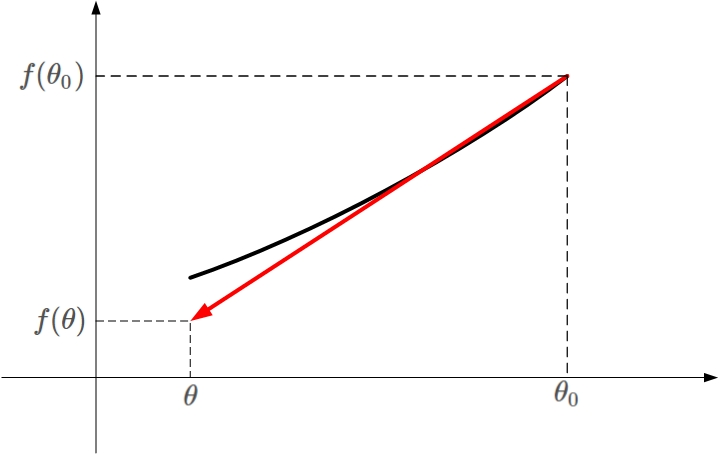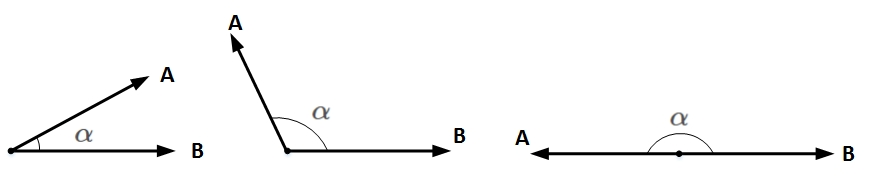# 为什么局部下降最快的方向就是梯度的负方向？## 什么是梯度？

=df(θ)dθ ∇ = d f ( θ ) d θ

## 梯度下降算法

θ=θ0ηf(θ0) θ = θ 0 − η ⋅ ∇ f ( θ 0 )

## 一阶泰勒展开式

f(θ)f(θ0)+(θθ0)f(θ0) f ( θ ) ≈ f ( θ 0 ) + ( θ − θ 0 ) ⋅ ∇ f ( θ 0 )f(θ)f(θ0)+(θθ0)f(θ0) f ( θ ) ≈ f ( θ 0 ) + ( θ − θ 0 ) ⋅ ∇ f ( θ 0 )

## 梯度下降数学原理

f(θ)f(θ0)+(θθ0)f(θ0) f ( θ ) ≈ f ( θ 0 ) + ( θ − θ 0 ) ⋅ ∇ f ( θ 0 )

θθ0=ηv θ − θ 0 = η v

f(θ)f(θ0)+ηvf(θ0) f ( θ ) ≈ f ( θ 0 ) + η v ⋅ ∇ f ( θ 0 )

f(θ)f(θ0)ηvf(θ0)<0 f ( θ ) − f ( θ 0 ) ≈ η v ⋅ ∇ f ( θ 0 ) < 0

vf(θ0)<0 v ⋅ ∇ f ( θ 0 ) < 0A A $A$$B$$B$均为向量， α α $\alpha$为两个向量之间的夹角。 A A $A$$B$$B$的乘积为：

AB=||A||||B||cos(α) A ⋅ B = | | A | | ⋅ | | B | | ⋅ c o s ( α )

||A|| | | A | | $||A||$ ||B|| | | B | | $||B||$均为标量，在 ||A|| | | A | | $||A||$ ||B|| | | B | | $||B||$确定的情况下，只要 cos(α)=1 c o s ( α ) = − 1 $cos(\alpha)=-1$，即 A A $A$$B$$B$完全反向，就能让 A A $A$$B$$B$的向量乘积最小（负最大值）。

v=f(θ0)||f(θ0)|| v = − ∇ f ( θ 0 ) | | ∇ f ( θ 0 ) | |

θ=θ0ηf(θ0)||f(θ0)|| θ = θ 0 − η ∇ f ( θ 0 ) | | ∇ f ( θ 0 ) | |

θ=θ0ηf(θ0) θ = θ 0 − η ∇ f ( θ 0 )

## 总结09-112724
01-289881
10-28475
05-241797
07-261655
09-02642
02-151809
09-212197
01-133115
03-24396
07-159515
06-01335
06-13155
10-051837
01-201318
01-183192
04-10281
02-021万+
05-15431

### “相关推荐”对你有帮助么？

•非常没帮助
•没帮助
•一般
•有帮助
•非常有帮助被折叠的  条评论 为什么被折叠?到【灌水乐园】发言¥2 ¥4 ¥6 ¥10 ¥20余额支付 (余额：-- )扫码支付获取中扫码支付点击重新获取扫码支付1.余额是钱包充值的虚拟货币，按照1:1的比例进行支付金额的抵扣。
2.余额无法直接购买下载，可以购买VIP、C币套餐、付费专栏及课程。余额充值